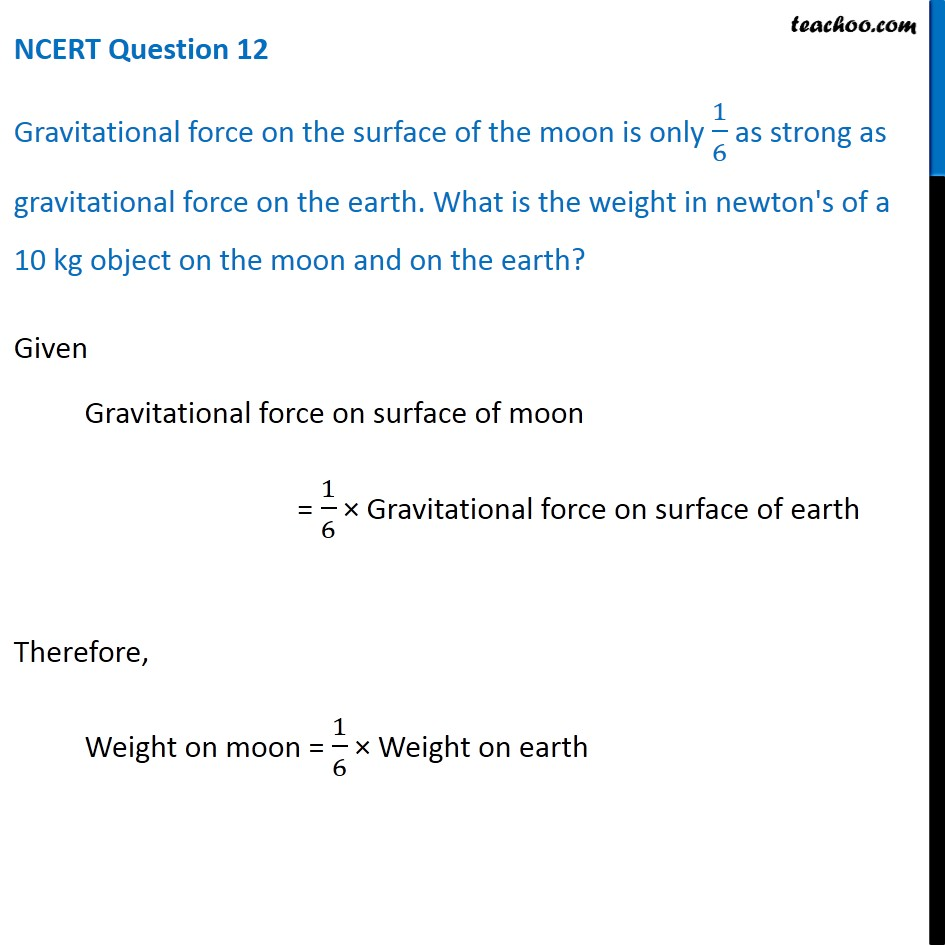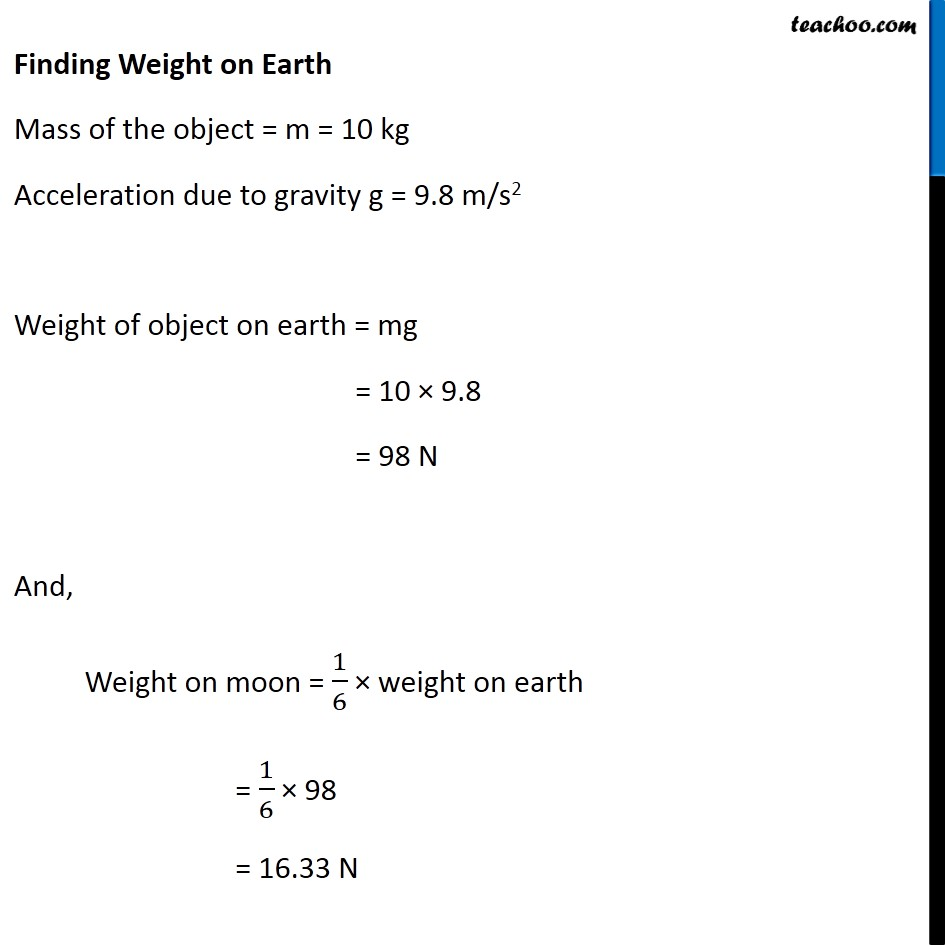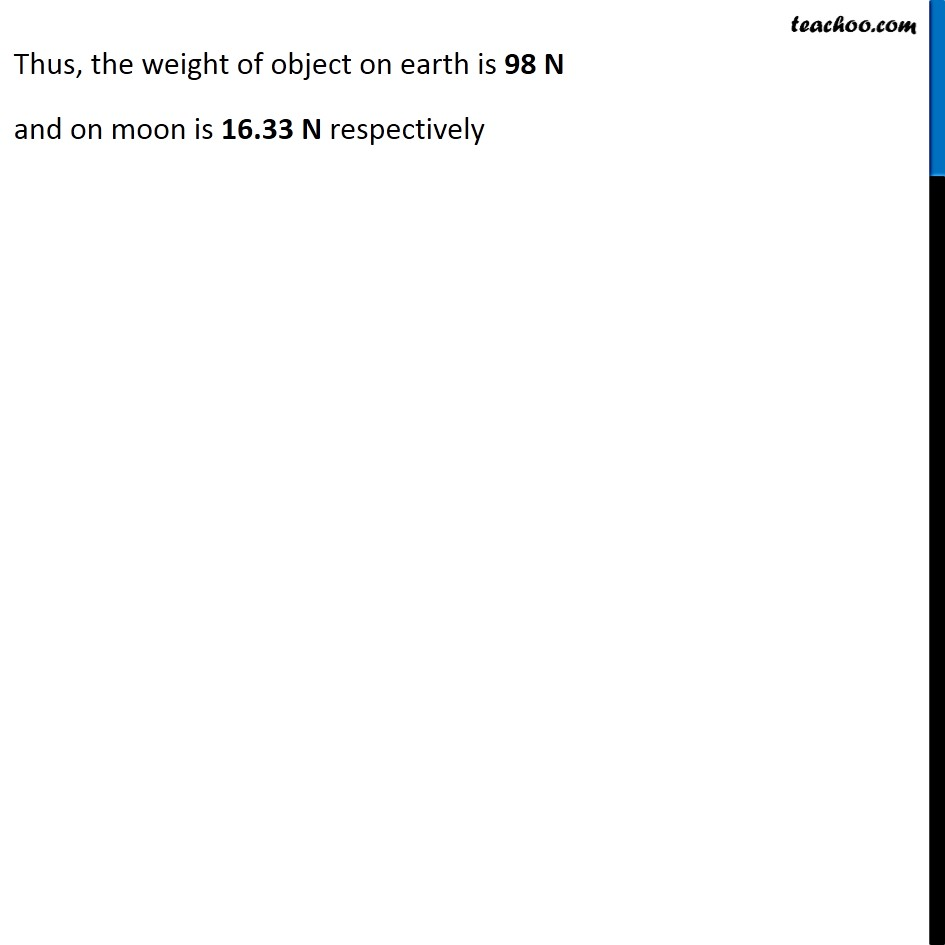NCERT Questions

Class 9
Chapter 10 Class 9 - GravitationGet live Maths 1-on-1 Classs - Class 6 to 12

### Transcript

NCERT Question 12 Gravitational force on the surface of the moon is only 1/6 as strong as gravitational force on the earth. What is the weight in newton's of a 10 kg object on the moon and on the earth? Given Gravitational force on surface of moon = 1/6 × Gravitational force on surface of earth Therefore, Weight on moon = 1/6 × Weight on earth Finding Weight on Earth Mass of the object = m = 10 kg Acceleration due to gravity g = 9.8 m/s2 Weight of object on earth = mg = 10 × 9.8 = 98 N And, Weight on moon = 1/6 × weight on earth = 1/6 × 98 = 16.33 N Thus, the weight of object on earth is 98 N and on moon is 16.33 N respectively